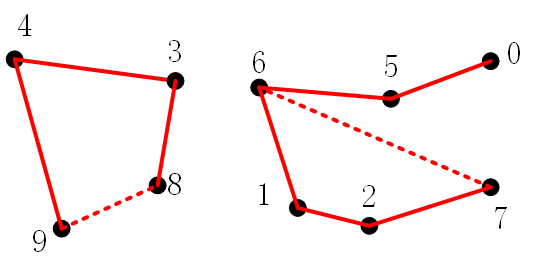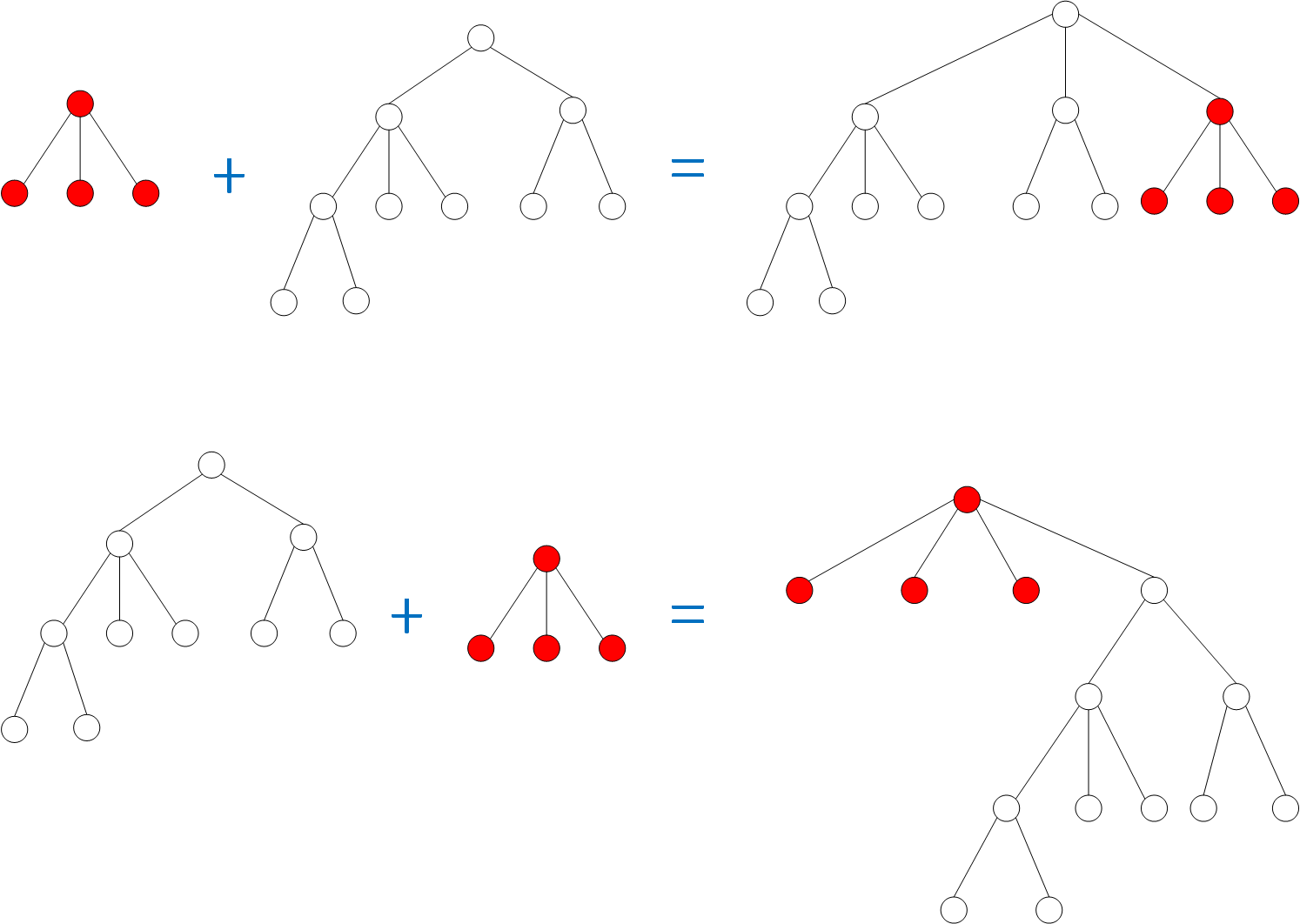# 并查集(Union-Find)算法详解

## 动态连通性element 0 1 2 3 4 5 6 7 8 9
group number 0 1 2 3 4 5 6 7 8 9

element 0 1 2 3 4 5 6 7 8 9
group number 0 1 2 3 3 5 6 7 8 9

## Quick-Find 算法

def con_eleGroupNum(eleList):
"""
construct the element-group number map
:param eleList: the list of distinct elements
:return: the dictionary with the form {element: group number}
"""
result = {}
num = 1
for i in eleList:
result[i] = num
num += 1
return result

def change_GroupNum(pairGroupNum, eleGroupNum):
"""
connect
:param pairGroupNum: the two group numbers of the pair of elements
:param eleGroupNum: the dictionary with the form {element: group number}
:return: None
"""
newGroupNum = min(pairGroupNum)
for i in eleGroupNum:
if eleGroupNum[i] == pairGroupNum or eleGroupNum[i] == pairGroupNum:
eleGroupNum[i] = newGroupNum

def quick_find(elePair, eleGroupNum):
"""
find and connect
:param elePair: the pair of elements
:param eleGroupNum: the dictionary
:return: None
"""
pairGroupNum = (eleGroupNum[elePair], eleGroupNum[elePair])
change_GroupNum(pairGroupNum, eleGroupNum)

## Quick-Union 算法

• 初始化：每个点看做一棵树，当然这是一棵只有根节点的树，存储了这个节点本身的值作为组别（你也可以令其他不会产生冲突的记号做组别）；

• 查询：对于点对(a,b)$(a, b)$，通过a$a$b$b$向其根节点回溯（当然初始时就是它们本身），判断其所在组别；

• 合并：若不在同一组别，令其中一个点（比如a$a$吧）所在树的根节点成为另一个点（比如b$b$）的根节点的孩子。这样即便再查询到a$a$，通过上面的查询过程，程序也会最终判断得到的是现在b$b$的根节点所在的组别，相当于是改变了a$a$所在组的全部元素的组别；

### 大树小树的合并技巧### 代码展示

class UFTreeNode(object):
def __init__(self, num):
# the group number
self.num = num

# its children
self.children = []

# its parent
self.parent = None

# the number of nodes that rooted by this node
self.weight = 1

def genNodeList(eleList):
"""
generating the node of each element
:param eleList: the list of elements
:return: a dictionary formed as {element: corresponding node}
"""
result = {}
for ele in eleList:
result[ele] = UFTreeNode(ele)
return result

def locPair(elePair, eleNodeMap):
"""
locate the positions of the pair of elements
:param elePair:
:param eleNodeMap: a dictionary formed as {element: corresponding node}
:return: the two nodes of the pair of elements
"""

return [eleNodeMap[elePair], eleNodeMap[elePair]]

def backtracking(node):
"""
1. find the root of a node
2. cut down the height of the tree
:param node:
:return: the root of node
"""
root = node

while root.parent:
cur = root
root = root.parent

# the grandfather node of cur exists
if cur.parent.parent:

# make the father of cur is its grandfather
grandfather = cur.parent.parent
grandfather.children.append(cur)

return root

def quickUnion(elePair, eleNodeMap):
"""
union process
:param elePair:
:param eleNodeMap:
:return:
"""
nodePair = locPair(elePair, eleNodeMap)

root_1, root_2 = backtracking(nodePair), backtracking(nodePair)

# if the two elements of the pair are not belongs to the same root (group)
if root_1 is not root_2:

if root_1.weight >= root_2.weight:

# update weight
root_1.weight += root_2.weight

# make the root2 as a subtree of root1
root_1.children.append(root_2)

# update the group number of root2
root_2.num = root_1.num

else:
root_2.weight += root_1.weight
root_2.children.append(root_1)
root_1.num = root_2.num